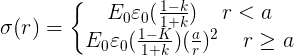# A dielectric hemisphere

sentinel

## Homework Statement

we put a dielectric hemishphere with radius (a) and dielectric coeeficient(k) on an infinite conducting plane.the system(hemsiphere and the plane) are in a constant electric field E0.
find the surface charge distrubution on the plane as a function of (r).
and explain what happens?

## Homework Equations

E in =1/k E (in a dielectric E weakens)
maxwell equations
e0E=surface charge(charge per area)

## The Attempt at a Solution

for the case k=1 there won't be any charge on the plane(I think)
also I tried to think what actually happens?will there be any charge inside the hemisphere?
and these are (I think) the solutions:Last edited by a moderator:

sentinel
anyone??

Staff Emeritus
Homework Helper
Gold Member

## Homework Statement

we put a dielectric hemisphere with radius (a) and dielectric coefficient(k) on an infinite conducting plane.the system(hemisphere and the plane) are in a constant electric field E0.
find the surface charge distribution on the plane as a function of (r).
and explain what happens?
What coordinate is r ?

## Homework Equations

E in =1/k E (in a dielectric E weakens)
Maxwell's equations
e0E=surface charge(charge per area)

## The Attempt at a Solution

for the case k=1 there won't be any charge on the plane(I think)
There is a charge on the plane, if the electric field is perpendicular to the plane.

Use Gauss's Law.

also I tried to think what actually happens?will there be any charge inside the hemisphere?
and these are (I think) the solutions:Homework Helper
Gold Member

## The Attempt at a Solution

for the case k=1 there won't be any charge on the plane(I think)
also I tried to think what actually happens?will there be any charge inside the hemisphere?
and these are (I think) the solutions:Shouldn't k = 1 correspond to a vacuum (i.e., no hemisphere present)? Then the field should just equal Eo everywhere.

I don't know your background, but you can solve this problem as a boundary value problem for the electric potential. Then use the potential to get the field at the surface of the plane, and hence the charge density.

You can also relate this problem to the problem of a dielectric sphere placed in an external uniform field with no conducting plane.